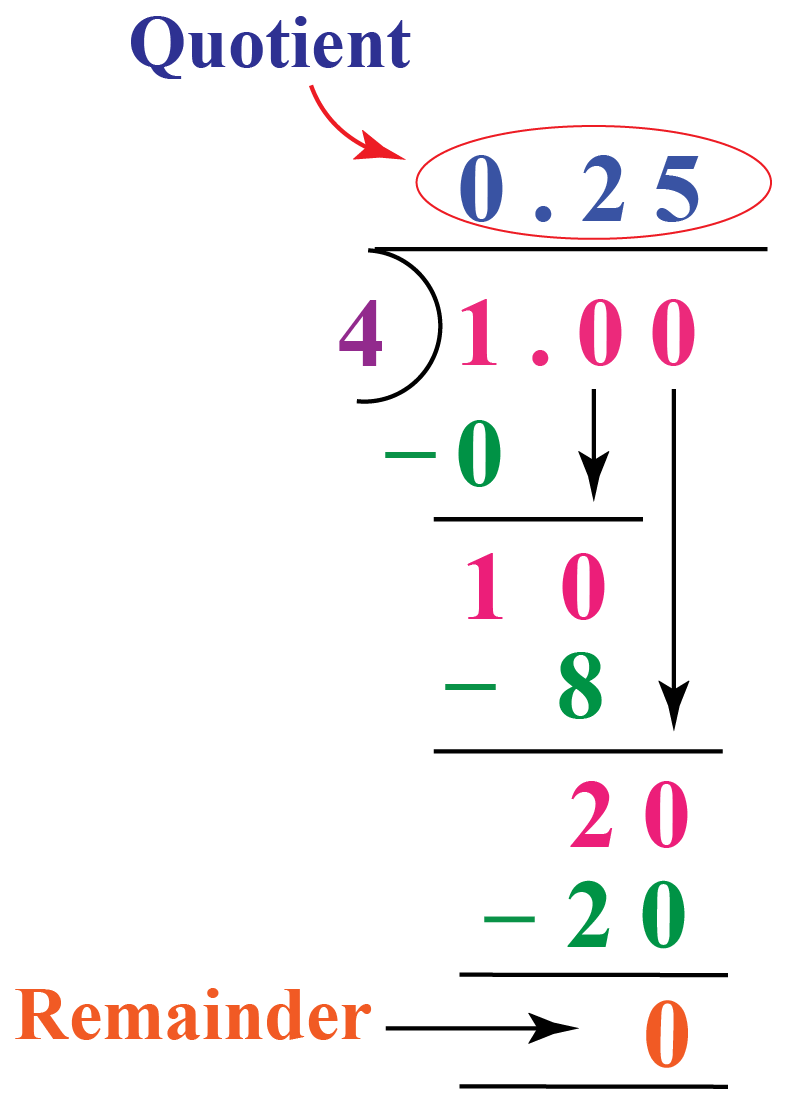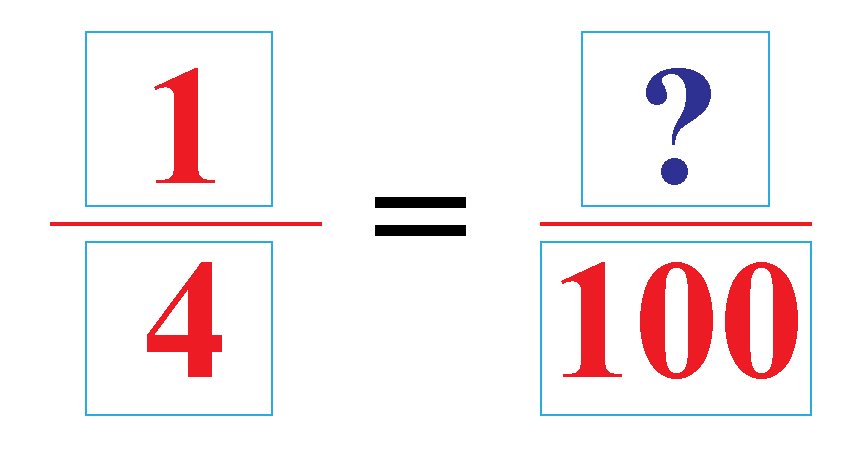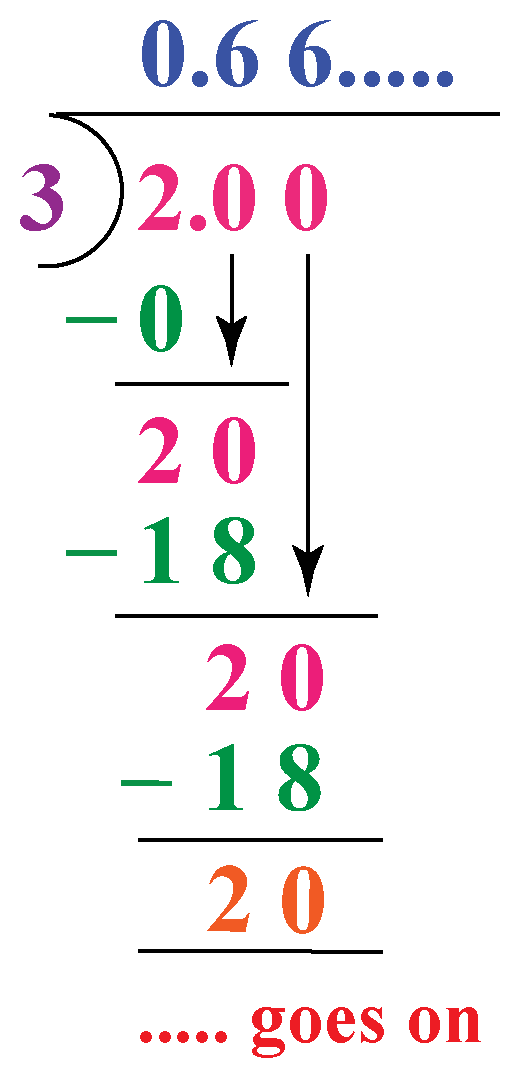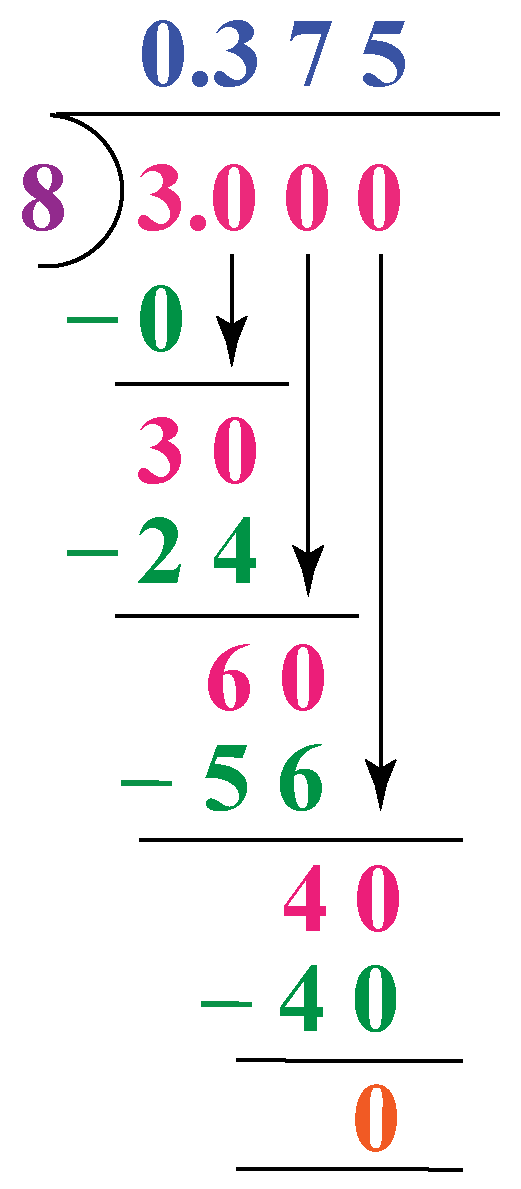# 1/4 As A Decimal

1/4 As A Decimal

Four friends, Rocky, Rooney, David, and Sam divided a donut equally among themselves. Sam asked, "What portion of the donut do I have?" Rocky replied, " One fourth." Sam asked again, "Is it possible to write it in a form that has denominator 1?".

Let us help Sam resolve his query in mere few minutes.

This section focuses on representing $$\frac{1}{4}$$ as a decimal along with some interactive examples and questions on the representation of $$\frac{3}{8}$$ as a decimal, $$\frac{2}{3}$$ as a decimal, and decimal to fraction conversion. Do not forget to try practice questions at the end of the page for a quick fun revision.

## Lesson Plan

 1 What is 1/4 as a Decimal? 2 Important Notes on 1/4 as a Decimal? 3 Think Out of Box! 4 Solved Examples on 1/4 as a Decimal 5 Interactive Questions on 1/4 as a Decimal

## What is 1/4 as a Decimal?

When we convert a fraction into decimal form, we convert it into a number that has denominator 1. So the numerator takes a form that has a decimal in it.

The decimal form of $$\dfrac{1}{4}\,$$ is 0.25

Let's see how to get that.

## How to change ¼ as a decimal

The fraction $$\dfrac{1}{4}\,$$can be converted to a form with denomiator 1 by finding its decimal form.

To get the decimal form of $$\dfrac{1}{4}\,$$, different methods can be used.

### Method 1

In this method, we use long division.

Here, Numerator = 1 and Denominator = 4. Let's divide 1 by 4So,

$$\dfrac{1}{4}=0.25.....\text{Decimal form}$$

We can use this method for converting any fraction to decimal form.

### Method 2

The other method is to convert the fraction into its equivalent fraction with denominator as a power of 10

The first power of 10 is 10 which is not a multiple of 4. So, let's look at the second multiple, 100, which is a multiple of 4

In the fraction $$\dfrac{1}{4}$$, denominator = 4\begin{align} \dfrac{1}{4}&=\dfrac{1\times 25}{4\times 25}\\&=\dfrac{25}{100}\end{align}

Now, observe that there are 2 zeros in the denominator.

So, there will be two digits after decimal in the numerator.

$$\dfrac{1}{4}=0.25$$

Similarly, if we have the fraction $$\dfrac{3}{8}$$, we can convert it into decimal by converting it into its equivalent fraction with denominator 1000

\begin{align} \dfrac{3}{8}&=\dfrac{3\times 125}{8\times 125}\\&=\dfrac{375}{1000}\\&=0.375\end{align}

We can even convert a decimal to fraction. For this, we consider the number of digits after the decimal.

Example: Take 0.65. There are two digits after the decimal.

\begin{align} 0.65&= \dfrac{65}{100}\\&=\dfrac{13}{20}\end{align}

 Example 1

Harry is struggling to express $$\dfrac{2}{3}\,$$ as decimal. Can you help him by using long division method to convert a fraction to decimal?

Solution

As denominator is 3, which is not a factor of 100, we will convert $$\dfrac{2}{3}\,$$ as a decimal by long division.The quotient is 0.6666.....= $$0.\overline{6}\cdot\cdot$$Digit 6 is recurring here as the remainder is going on repeating.

So, we round off 0.6666..... to 0.67 (rounding off upto 2 decimal places).

 $$\therefore\dfrac{2}{3}=0.67$$
 Example 2

Mia wants to express $$\dfrac{3}{8}\,$$ as a decimal number using long division. Help her to reach the correct answer.

Solution

Mia needs to divide 3 by 8 and continue dividing till she gets the remainder 0$$\therefore$$Decimal form of $$\dfrac{3}{8}$$ is 0.375Think Tank

Find decimal form of fractions $$\dfrac{1}{5}$$ and $$\dfrac{1}{7}$$. What difference did you notice in their decimal forms?

(Hint: Observe the remainders in both the cases.)

## Interactive Questions

Here are a few activities for you to practice.

## Let's Summarize

We hope you enjoyed learning about 1/4 As A Decimal with the simulations and practice questions. Now, you will be able to easily solve problems on 3/8 as a decimal, 2/3 as a decimal, and decimal to fraction.

At Cuemath, our team of math experts is dedicated to making learning fun for our favorite readers, the students!

Through an interactive and engaging learning-teaching-learning approach, the teachers explore all angles of a topic.

Be it worksheets, online classes, doubt sessions, or any other form of relation, it’s the logical thinking and smart learning approach that we, at Cuemath, believe in.

## 1. What is $$\dfrac{1}{8}$$ as a decimal?

The decimal form of  $$\dfrac{1}{8}=0.125$$

## 2. What is $$\dfrac{9}{20}$$ as a decimal?

The decimal form of$$\,\dfrac{9}{20}=0.45$$

## 3. How do you write $$\dfrac{1}{3}$$ as a decimal?

$$\dfrac{1}{3}$$ can be written in decimal form as 0.333333....(never ending), which can be rounded off to 0.3

More Important Topics
More Important Topics
Learn from the best math teachers and top your exams

• Live one on one classroom and doubt clearing
• Practice worksheets in and after class for conceptual clarity
• Personalized curriculum to keep up with school